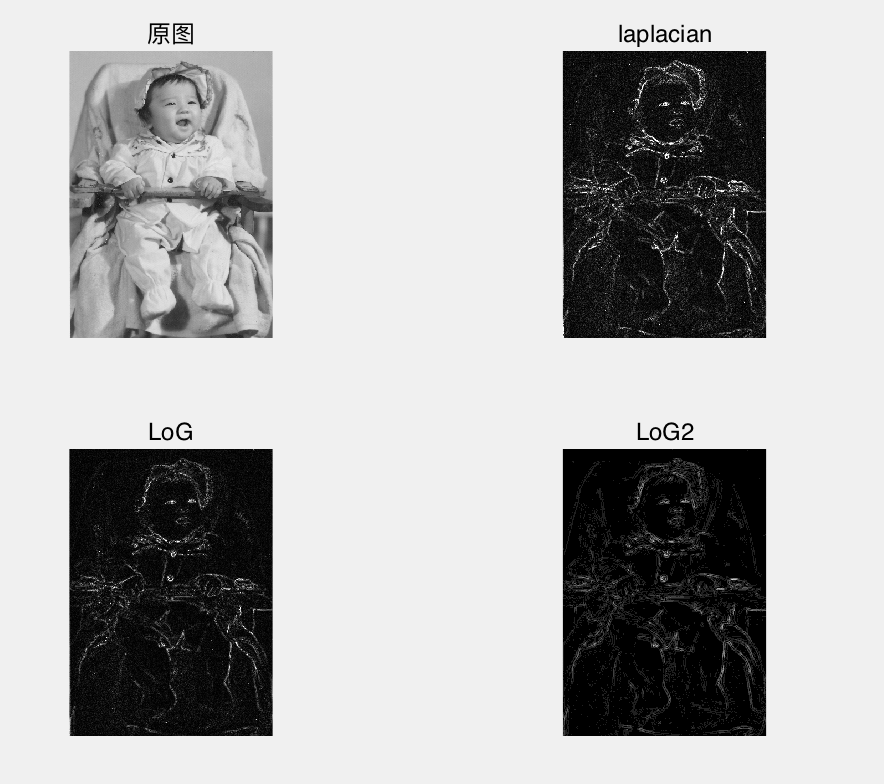# 中值滤波与图像锐化

Posted by jjx on December 23, 2016

• 中值滤波及其改进算法
• 图像锐化， 包括梯度算子、拉普拉斯算子、高提升滤波和高斯-拉普拉斯变换
• 本章的典型囊例分析
• 对椒盐噪声的平滑效果比较
• Laplacian与LoG算子的锐化效果比较

#### 中值滤波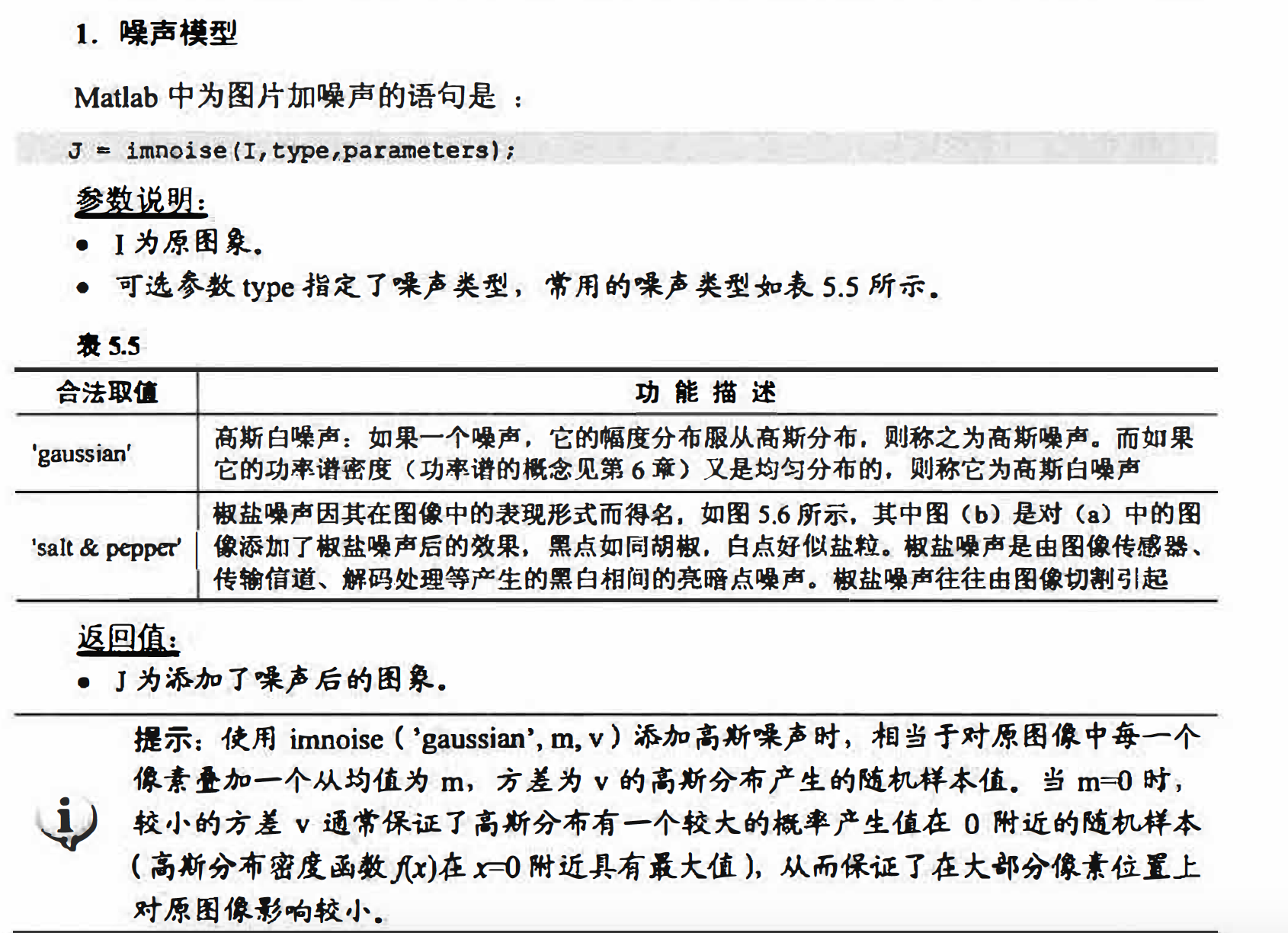Matlab提供了medfilt2函数实现中值滤波， 原型为:
I2 = medfilt2(I1,[m,n])

• I1是原因矩阵．
• m和n是中值滤波处理的模板大小，默认3x3.

I = imread('lena_salt.bmp');
J = imnoise(I,'salt & pepper');
w = [1,2,1;2,4,2;1,2,1]/16;
J1 = imfilter(J,w,'corr','replicate');

w = [1,1,1;1,1,1;1,1,1]/9;
J2 = imfilter(J,w,'corr','replicate');

J3 = medfilt2(J,[3,3]);
figure;
subplot(2,3,1);
imshow(I),title('原图像');
subplot(2,3,2);
imshow(J),title('椒盐噪声');
subplot(2,3,4);
imshow(J1),title('高斯平滑');
subplot(2,3,5);
imshow(J2),title('平均平滑');
subplot(2,3,6);
imshow(J3),title('中值平滑');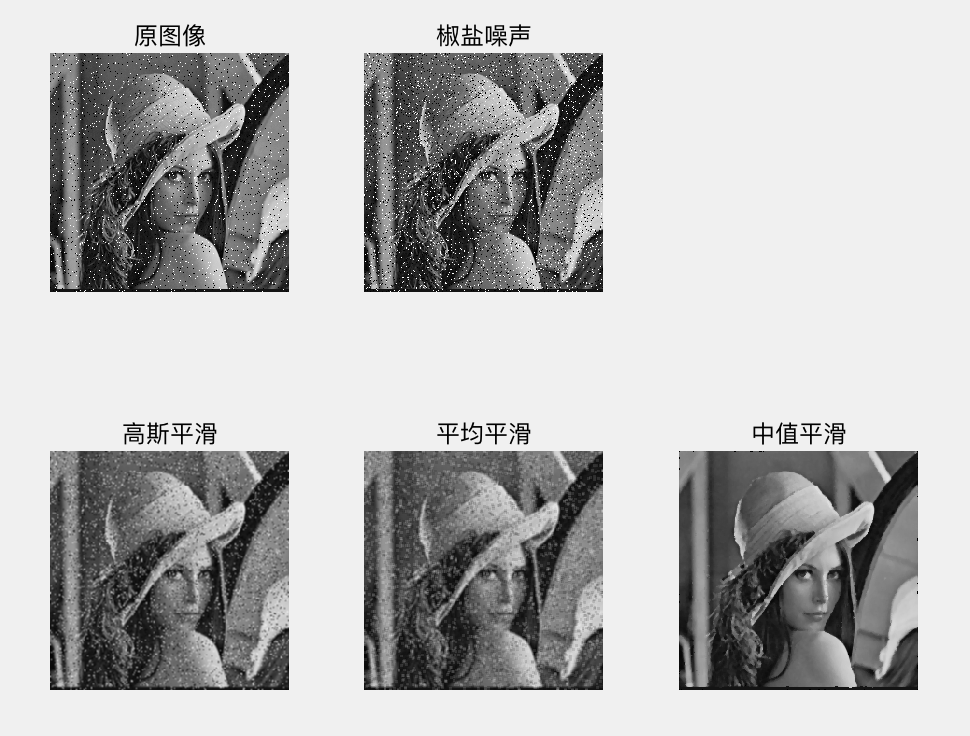### 图像锐化

#### 基于一阶导数的图像增强一一梯度算子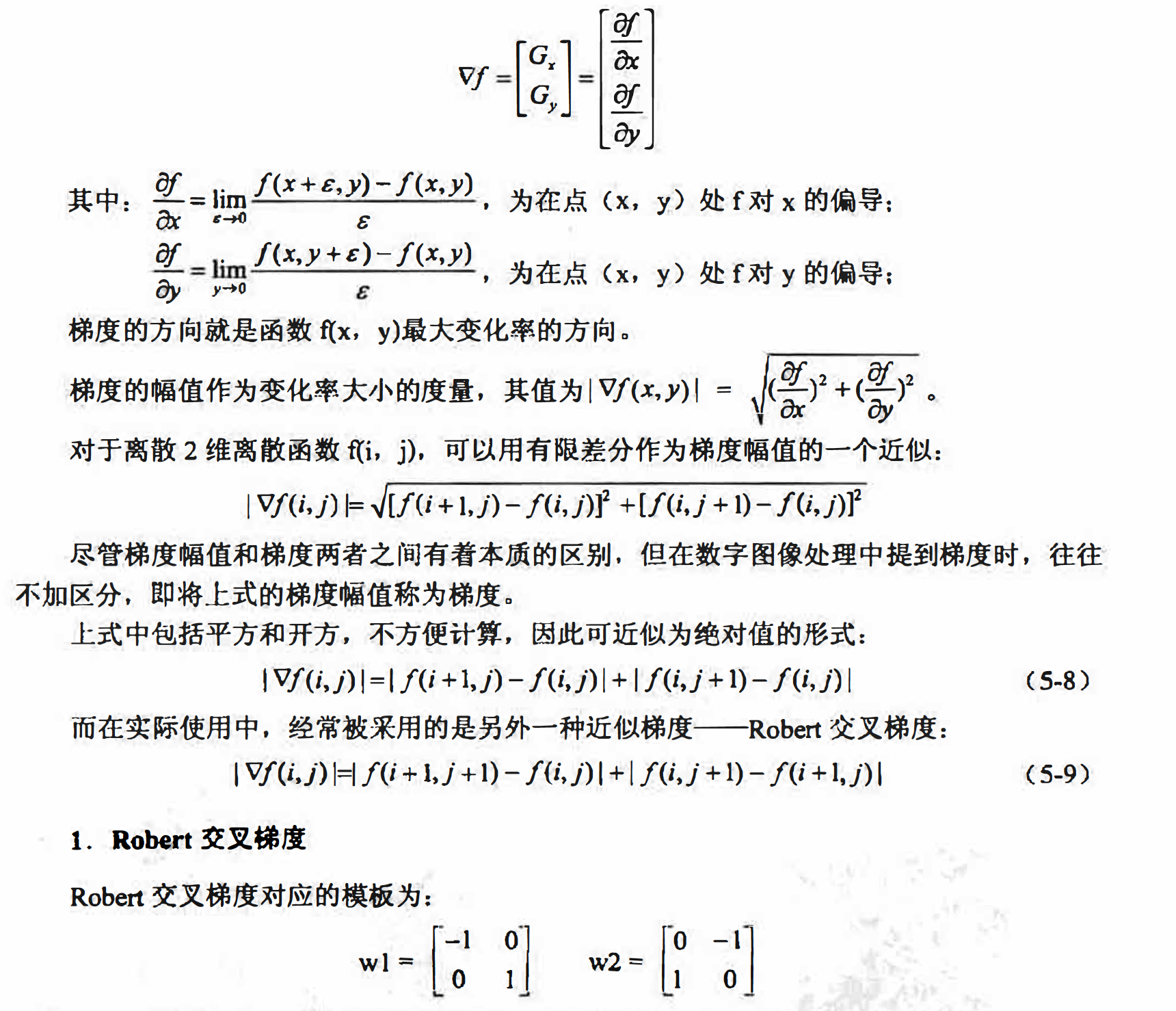其中，$w_1$对接近正45 度边缘有较强响应： $w_2$ 对接近负45 度边缘有较强响应．

I = imread('bacteria.BMP');
temp = I;
I = double(I);
w1 = [-1 0; 0 1];
w2 = [0 -1;1 0];

G1 = imfilter(I,w1,'corr','replicate');
G2 = imfilter(I,w2,'corr','replicate');
G = abs(G1)+abs(G2);
figure;
subplot(2,2,1);
imshow(temp),title('原图像');
subplot(2,2,2);
imshow(abs(G1),[]),title('w1滤波');
subplot(2,2,3);
imshow(abs(G2),[]),title('w2滤波');
subplot(2,2,4);
imshow(G,[]),title('Robert梯度');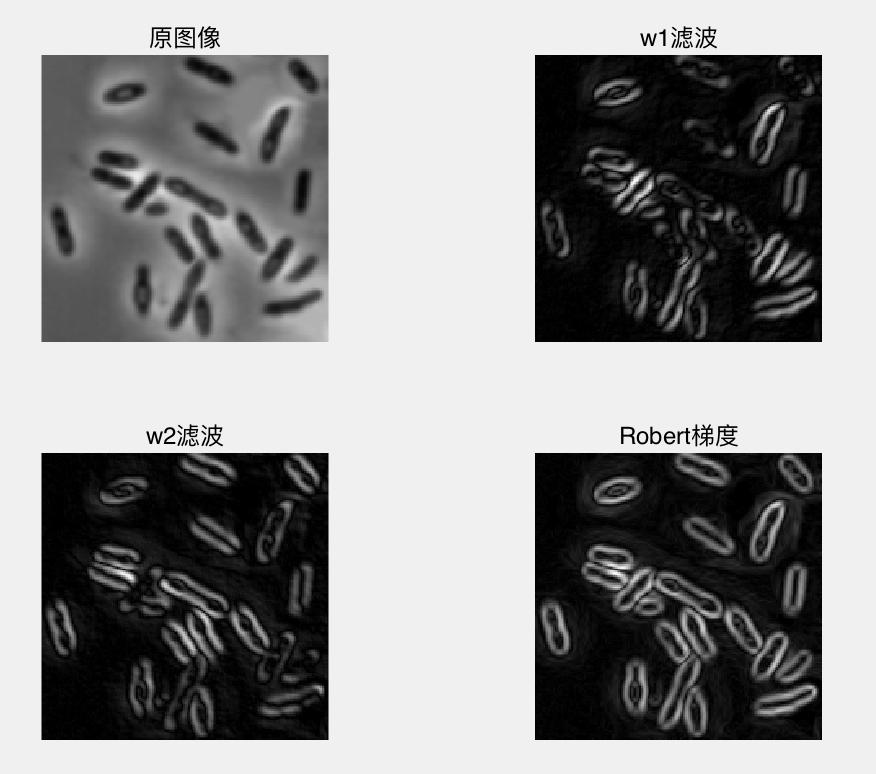imshow(K,[])显示K，并将K的最大值和最小值分别作为纯白(255)和纯黑(0)，中间的K值映射为0到255之间的标准灰度值。

#### Sobel梯度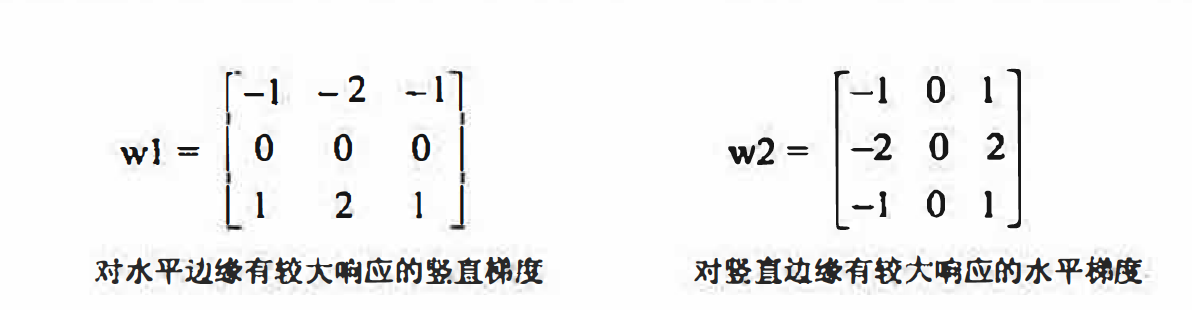下面的Matlab 程序计算了一幅图像的竖直和水平梯度， 它们的和可以作为完整的Sobel梯度。

I = imread('bacteria.BMP');
w1 = fspecial('sobel');
w2 = w1';
G1 = imfilter(I,w1);
G2 = imfilter(I,w2);
G = abs(G1)+abs(G2);
figure;
subplot(2,2,1);
imshow(I),title('原图像');
subplot(2,2,2);
imshow(G1,[]),title('水平sobel');
subplot(2,2,3);
imshow(G2,[]),title('竖直sobel');
subplot(2,2,4);
imshow(G,[]),title('sobel');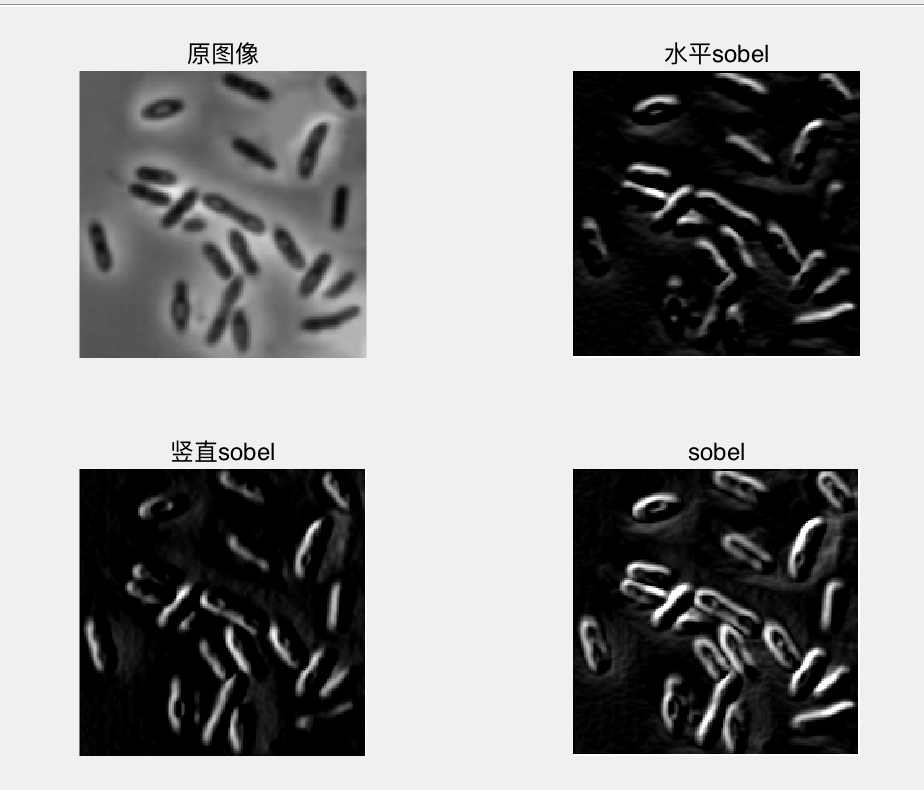#### 基于二阶微分的图像增强拉普拉斯算子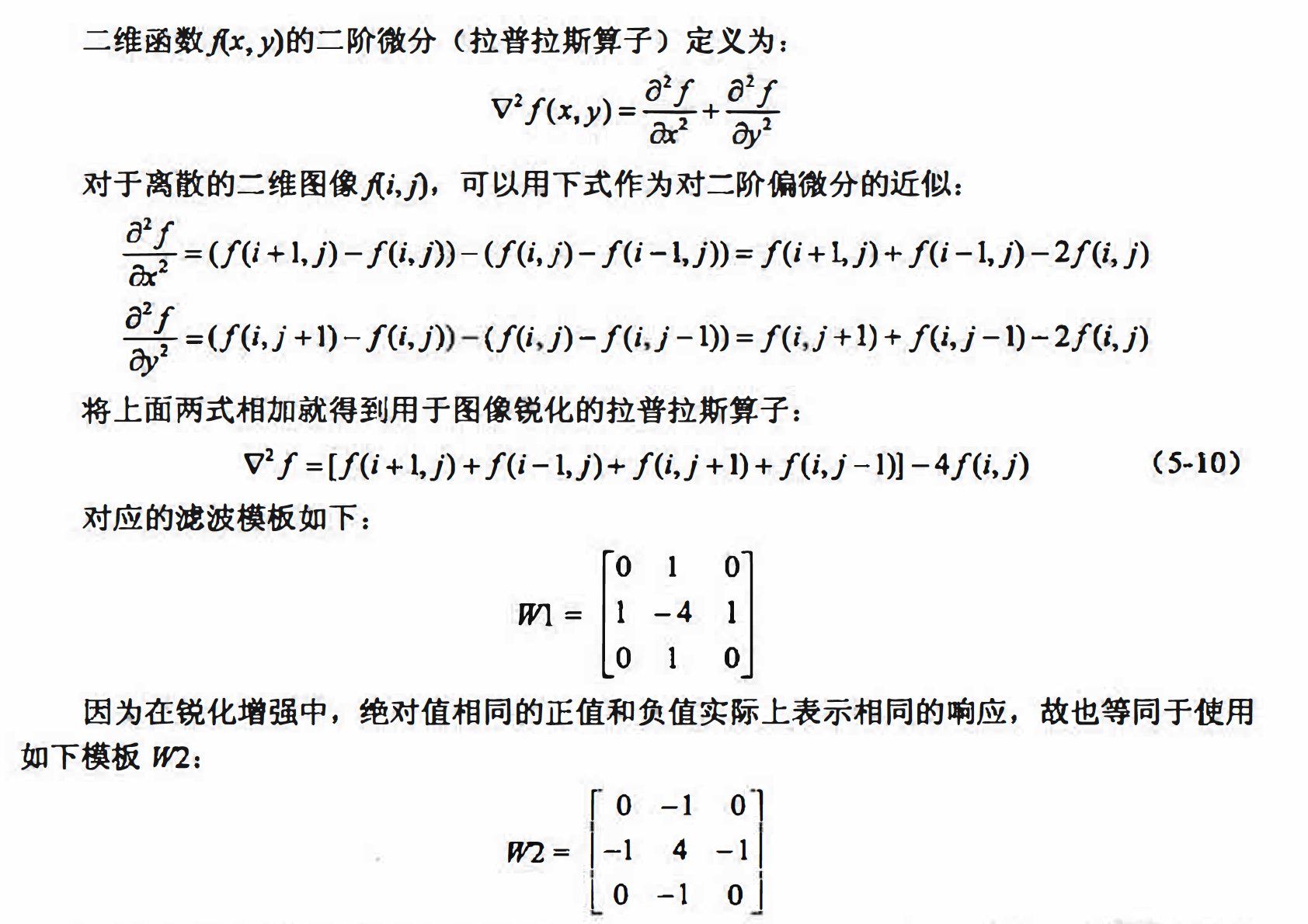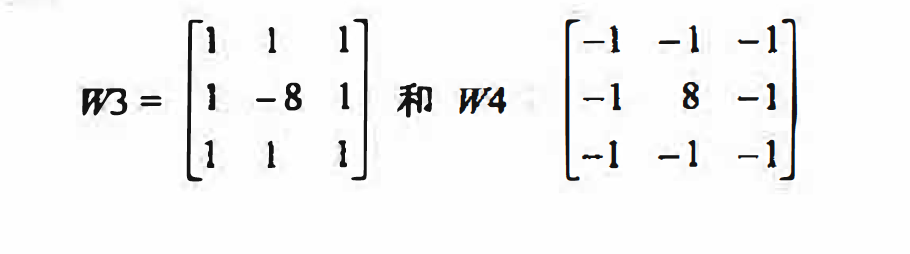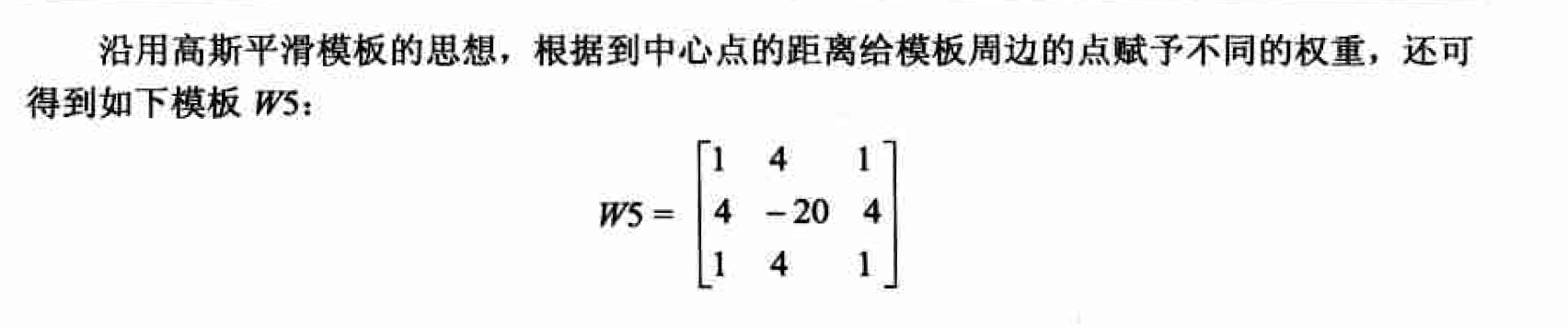I = imread('bacteria.BMP');
temp = I;
I = double(I);
w1 = [0 -1 0;-1 4 -1;0 -1 0];
L1 = imfilter(I,w1,'corr','replicate');
w2 = [-1 -1 -1;-1 8 -1 ;-1 -1 -1];
L2 = imfilter(I,w2,'corr','replicate');
w3 = [1 4 1;4 -20 4;1 4 1];
L3 = imfilter(I,w3,'corr','replicate');
figure;
subplot(2,2,1);
imshow(temp),title('原图像');
subplot(2,2,2);
imshow(abs(L1),[]);
subplot(2,2,3);
imshow(abs(L2),[]);
subplot(2,2,4);
imshow(abs(L3),[]);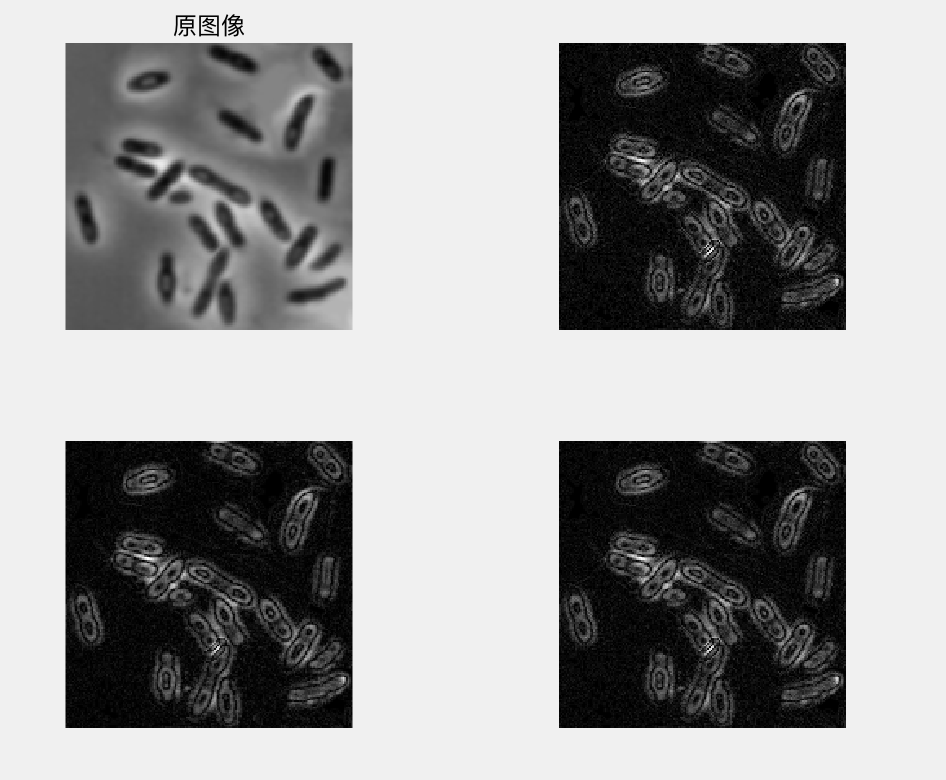#### 基于一阶与二阶导数的锐化算子的比较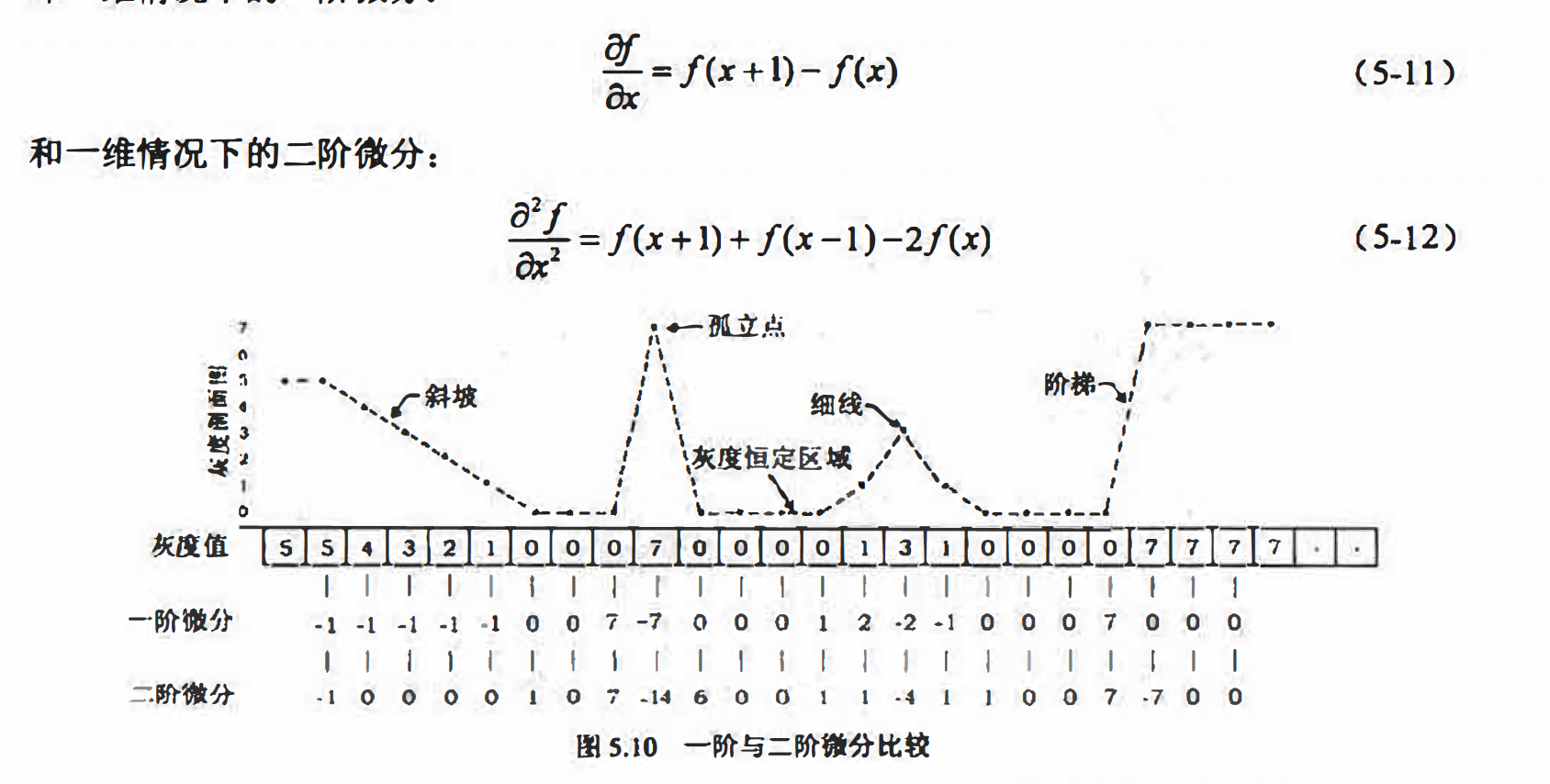• 一阶导数通常会产生较宽的边缘．
• 二阶导数对于阶跃性边缘中心产生零交叉，而对于屋顶状边缘（细线），二阶导数取极值．
• 二阶导数对细节有较强的响应， 如细线和孤立噪声点

5.5节的最后我们提到了平滑滤波器又可以称为低通滤波器，相应的，上面介绍的几种锐化滤波器也可以称为高通滤波器，得名的具体原因将在下一章“频域滤波”中说明．

#### 高提升滤波及其实现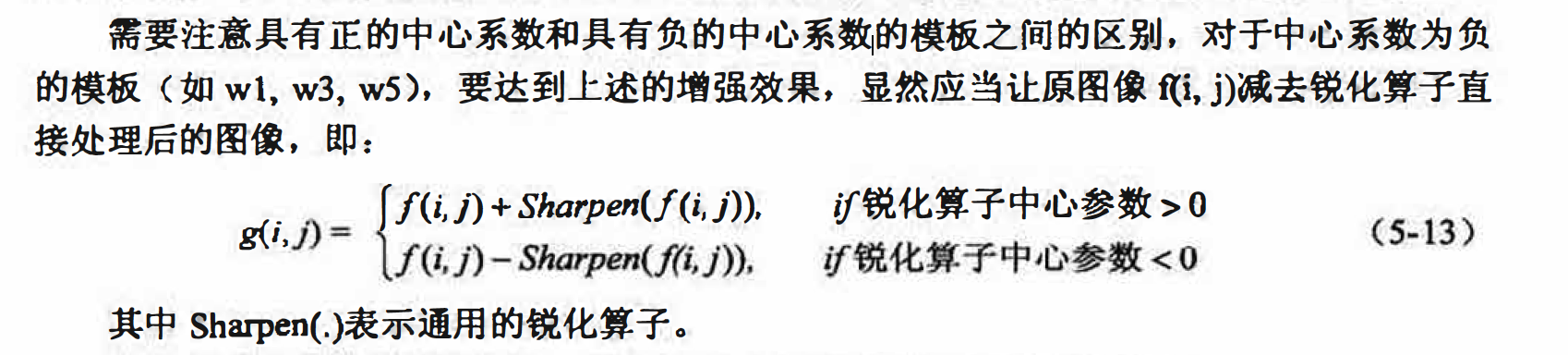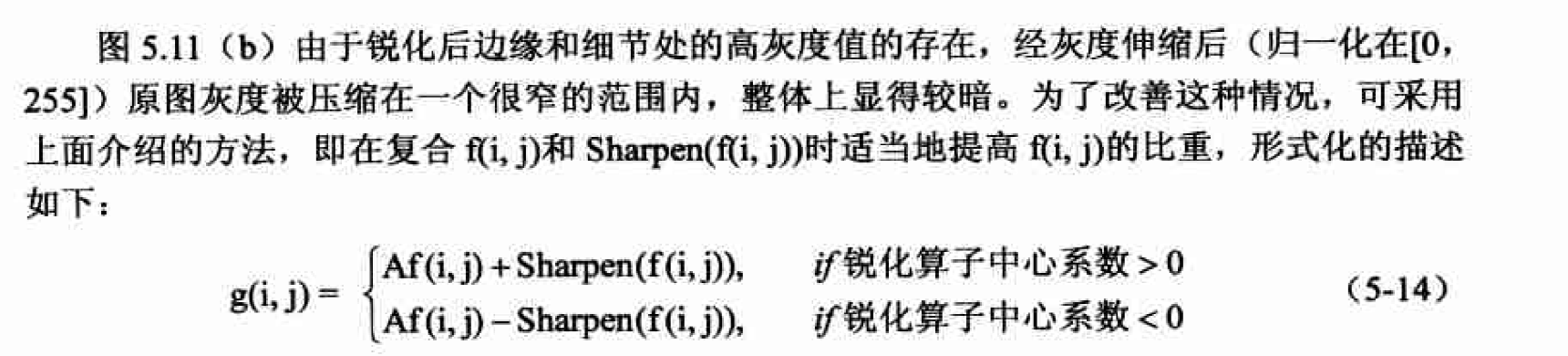形如式5-14这样的滤波处理就称为高提升滤波．

(1)图像锐化。
(2）原图像与锐化图像的按比例混合．
(3）混合后的灰度调整〈归一化至［0,255］。

#### 高斯·拉普拉斯交换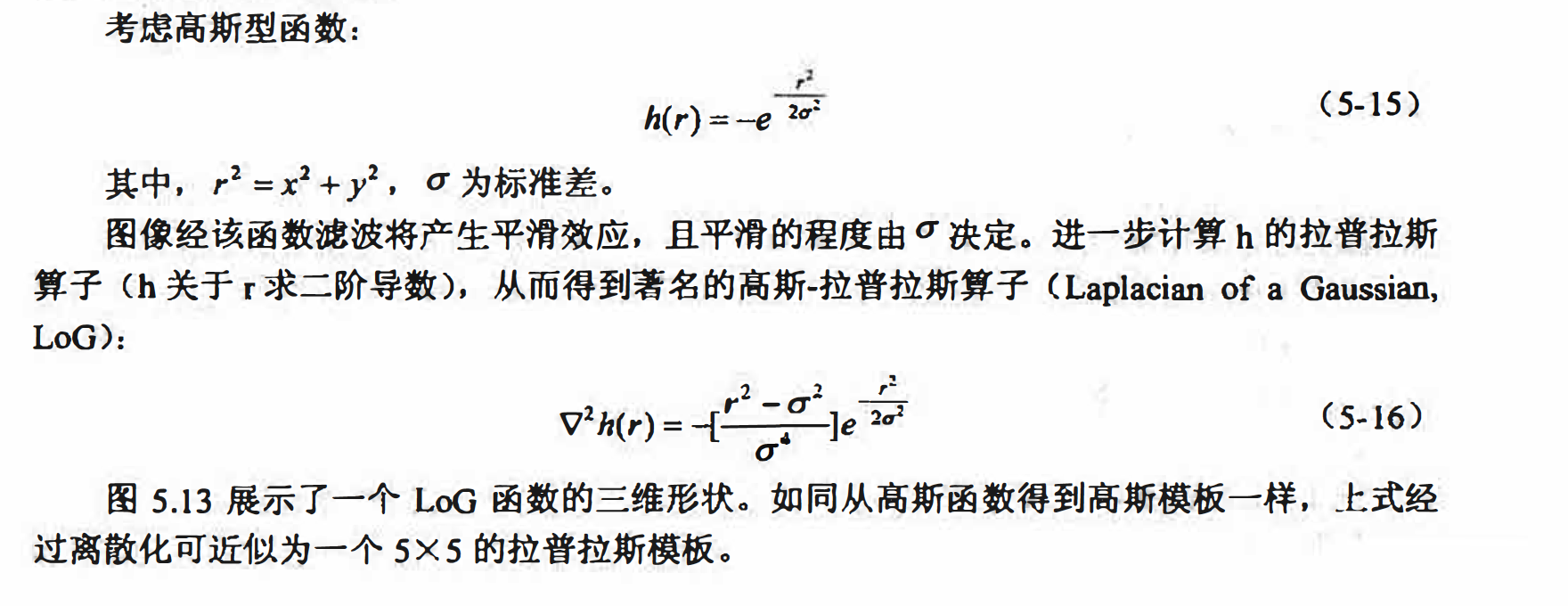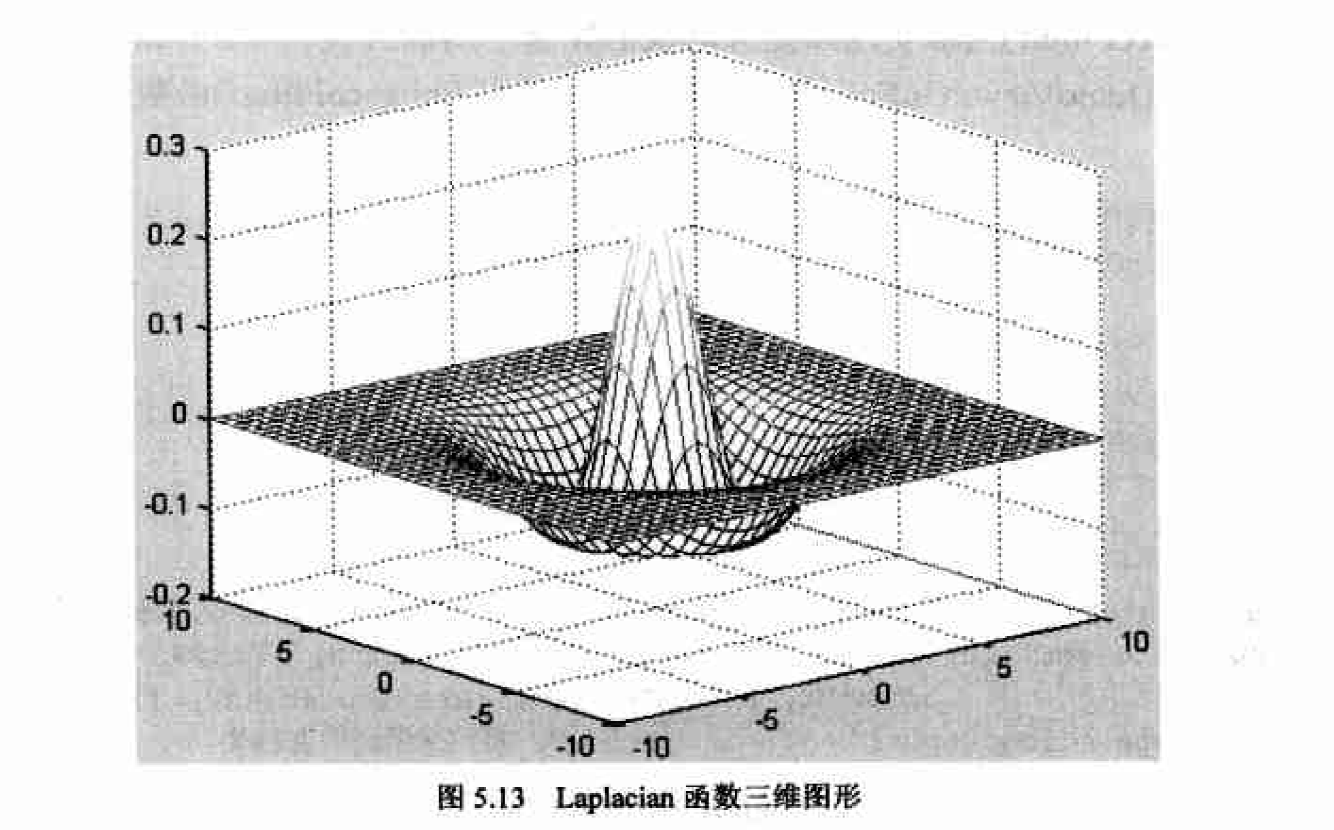Laplacian与LoG算子的锐化效果比较

I = imread('baby.bmp');
Id = double(I);
h_lap = [ -1 -1 -1;-1 8 -1;-1 -1 -1];
I_lap = imfilter(Id,h_lap,'corr','replicate');

h_log = fspecial('log',5,0.5);
I_log = imfilter(Id,h_log,'corr','replicate');
h_log2 = fspecial('log',5,2);
I_log2 = imfilter(Id,h_log2,'corr','replicate');

figure;
subplot(2,2,1);
imshow(I),title('原图');
subplot(2,2,2);
imshow(uint8(abs(I_lap)),[]),title('laplacian');

subplot(2,2,3);
imshow(uint8(abs(I_log)),[]),title('LoG');
subplot(2,2,4);
imshow(uint8(abs(I_log2)),[]),title('LoG2');